SCHOLASTIC APTITUDE TEST 2000

MATHEMATICS

 Time: Two Hours Max. Marks: 60

• Answers must be written in English or the medium of instruction of the candidate in High school.
• Attempt all questions.
• Answer all the questions in the booklets provided for the purpose. No pages should be removed from the booklets.
• There is no negative marking.
• Answer all questions of section I at one place. Same applies to section II. The remaining questions can be answered in any order.
• Answers to sections I and II must be supported by mathematical reasoning.
• Use of calculators, slide rule, graph paper and logarithmic, trigonometric and statistical tables is not permitted

Section I

This section has 5 questions. Each question is provided with five alternative answers. Only one of them is correct. Indicate the correct answer by A or B or C or D or E. Order of the questions must be maintained. (5x2=10 Marks)

1. A1A2………..A2000 is a polygon of 2000 sides. P is a point in the plane of the polygon which is equidistant from all the vertices of the polygon. Then
 A) There is no such P B) There is exactly one such P C) Either A or B D) The locus of P is a straight line E) The locus of P is a circle
1. Consider the following expressions in a,b,c.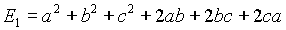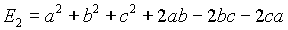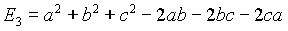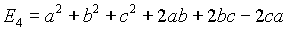1. The expressions which are not perfect squares among E1,E2,E3 and E4 are
 A) E1,E3 B) E2,E3 C) E2,E4 D) E1,E4 E) E3,E4
1. Three angles of a convex polygon measure each p /3 radians. Let n be the number of sides of the polygon. Then
 A) The hypothesis is not sufficient to evaluate n B) n=3 C) n=6 D) n=9 E) None of these
1. There are n rods,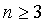. The ith rod has length 2i units. The number of closed convex polygonal frames that can be made by joining the rods end – to – end is
 A) 0 B) n C) n! D) nn E) 2n-1
1. Integers from 1 to 2000 are written. A single operation consists of cancelling any two of the numbers and replacing them by their product. After 1999 such operations
A) No number will be left
B) Precisely 2 numbers will be left
C) More than two numbers may be left
D) Only one number will be left and it is 2000!
E) Only one number will be left, but it cannot be uniquely determined

Section II

This section has 5 questions. Each question is in the form of a statement with a blank. Fill the blank so that the statement is true. Maintain the order of the questions. (5x2=10 Marks)

1. All possible 2000-gons are inscribed in a circle of perimeter p. Let X=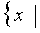an inscribed polygon has a side of length x.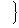. Then the greatest member of X is ____________ .
2.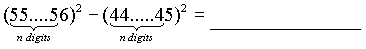3. Triangle ABCThe altitude through A has length h. is right angled at A. Then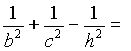4. p is a prime, n a positive integer and n+p =2000. LCM of n and p is 21879. Then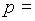______________ and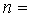.
5. The units digit of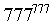is _________.

Section III

This section has 5 questions. The solutions are short and methods, easily suggested. Very long and tedious solutions may not get full marks. (5x2=10 Marks)

1. Does there exist a right angled triangle with integral sides such that the hypotenuse measures 2000 units of length?
2. If a,b,c are not all equal and (a+b+c)>0, determine the sign of a3+b3+c3-3abc.
3. If real numbers x,y,z satisfy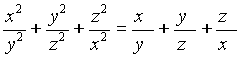, then prove that x=y=z
4. Write down the solutions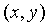of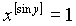,if x >0 and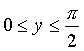and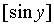denotes the integral part of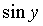5. Given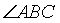and a point D in its interior. The problem is to find E on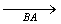and F on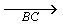such that D is the mid point of line segment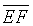. A student suggests the following method of construction: Join BD and extend it to B such that BD = DB. Draw parallel to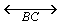through B. Take for E the point where this parallel cuts. Join ED and produce it to meet.Take for F this point of intersection.

Discuss the validity or otherwise of this method of construction.

Section IV

This section has 5 questions. The solutions involve either slightly longer computations or subtler approaches. Even incomplete solutions may get partial marks.

1. Consider the equation in positive integers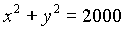with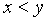.
a) Prove that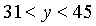.
b) Rule out the possibility that one of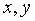is even and the other is odd.
c) Rule out the possibility that bothare odd.
d) Prove thatis a multiple of 4.
e) Obtain all the solutions. (10 Marks )
2. ABCD is a trapezium with AB and CD as parallel sides. The diagonals intersect at O. The area of the triangle ABO is p and that of the triangle CDO is q. Prove that the area of the trapezium is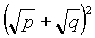. ( 5 Marks )
3. Manipulate the equality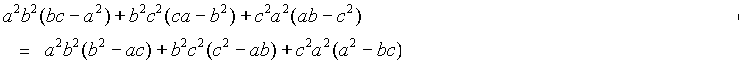until the equlity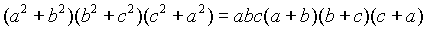is obtained ( 5 Marks )

1. If a triangle and a convex quadrilateral are drawn on the same base and no part of the quadrilateral is outside the triangle, show that the perimeter of the triangle is greater than the perimeter of the quadrilateral. ( 5 Marks )
2. If a line parallel to, but not identical with, x- axis cuts the graph of the curve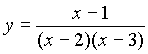at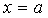,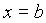, then evaluate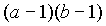( 5 Marks )